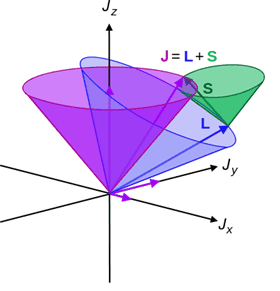# The average value of S operator

• B
Viona
TL;DR Summary
Why the average value of S operator is considered to be the projection of S onto J ?
While reading in the book of Introduction to Quantum Mechanics by David Griffith in the section of Fine structure of Hydrogen: spin- orbit coupling, he said that the average value of S operator is considered to be the projection of S onto J. I could not understand why he assumed that. please help me to understand.Gold Member
Given the fine-structure Hamiltonian ##H = A\vec{L} \cdot \vec{S}##, try to calculate the commutator ##[H,\vec{S}]##. What do you get, in vector form?

•Viona
What I was getting at is that if you do the algebra, I get $$[H,\vec{S}] = iA\vec{S} \times \vec{L} = iA\vec{S} \times \vec{J}$$ where the last step is true because ##\vec{J} = \vec{L} + \vec{S}##. Use the Ehrenfest theorem on this to get $$\frac{d\langle \vec{S} \rangle}{dt} = \frac{A}{\hbar} \langle \vec{S} \times \vec{J} \rangle$$
•